# Network properties and a Counterexample

Tom Kelliher, CS 315

Feb. 5, 1999

### Announcements

Will assign homework Monday.

### From Last Time

Linear array sorting example, network properties.

### Outline

1. Network (graph) properties.

2. A lower bound counterexample.

3. Properties of our fixed-network connection model.

# Lower Bound Arguments

The following are tools we use to establish lower bounds on problems and, hence, show that a particular parallel algorithm is optimal. As we will see, they must be applied with care.

Assume the bit model, so we have an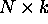sorting network in the following.

1. I/O bandwidth.

What is the I/O bandwidth of the sorting network? This makes sorting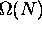.

2. Diameter of the network: Defined as the maximum distance between two vertices in the graph.

What is the diameter of the sorting graph?

Can we construct a sorting problem that will force the two most distant cells to communicate?

What's the lower bound from this perspective?

3. Bisection width of the network: Defined as the number of edges which must be cut to split the graph in ``half.''

What is the bisection width of the sorting graph?

Can we construct a sorting problem that will force all the data to cross this boundary?

What's the lower bound from this perspective?

# A Counterexample

Consider sorting within a complete binary tree: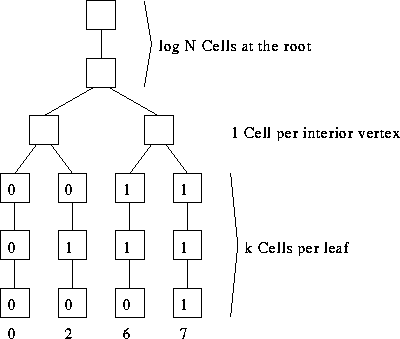1. Assumption: Allbits appear simultaneously.

2. I/O bandwidth? Diameter? Bisection width?

3. Lower bound for sorting on a binary tree?

4. Almost right. Holds for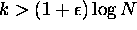.

5. So, how do we beat the lower bound? By counting.

## Example: k=1

1. Problem of unary to binary conversion.

2. ``Easy'' if interior cells are word processors.

3. Each interior processor need only be a serial adder and we do bit-wise serial addition with numbers transmitted lsb-first.

Example: Bit-wise addition of 0110 and 0011.

4. Example: counting the 1's when N = 8.

5. Setting the leaf bits:
1. Assume we have m 1's.

2. Set the rightmost m leaves. Clear the leftmost N - m leaves.

3. Observation: right 0 leaf is numbered N - 1 - m.

This is the bit-wise complement of m.

4. Use N - 1 - m to find a path to that leave, clearing leaves to the left, setting leaves to the right, and clearing that leave.

# Properties of the Fixed-Connection Network Model

1. Processor properties:
1. Local control.

2. Computation is a function of local storage and local inputs.

3. Variations. Processor might know such things as its address, topology/size of the network, time.

Storage is usually constant, but may vary with size of network.

4. A bit processor may perform a constant number of bit operations per step. Similarly for a word processor.

5. Limits on word size usually.

6. Packets: indivisible communication data stamped with a key.

2. Interconnection properties:
1. Connections may not vary with time.

2. A constant amount of communication (bits, words, packets, as appropriate) can occur across a connection per step.

3. Bounded-degree network. Sometimes degree will be O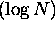.

Size is polynomial in N.

3. I/O protocol properties:
1. Each input is provided only once.

2. I/O is when and where oblivious: the time and location, for each piece of data, of I/O must be specified in advance.

Without this restriction, we could sort in constant time.

4. Comment: This model describes systolic VLSI very well. It doesn't describe general VLSI well since we don't have ``long'' wires. But, how does one model the communication properties of a long wire?

Thomas P. Kelliher
Fri Feb 5 10:06:30 EST 1999
Tom Kelliher